Publicité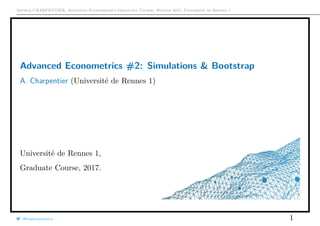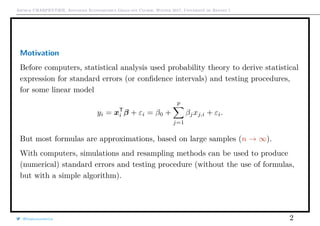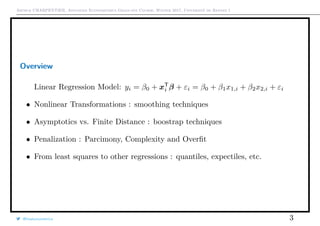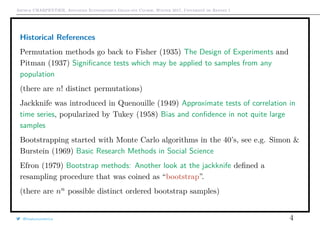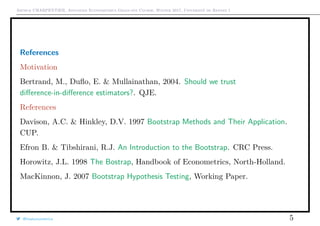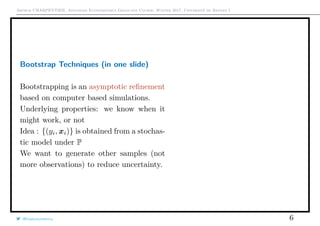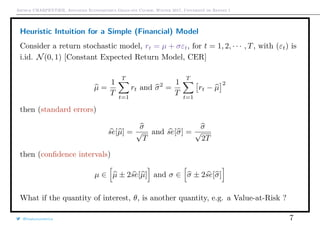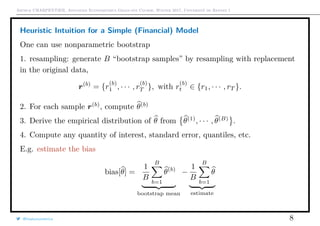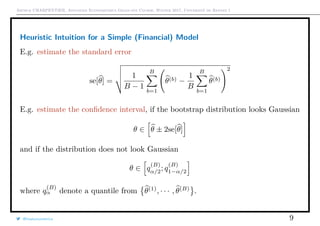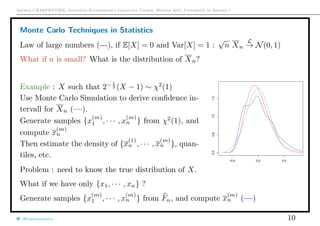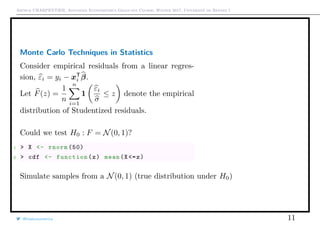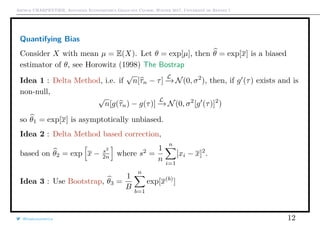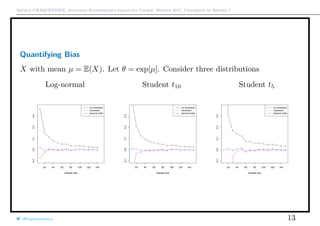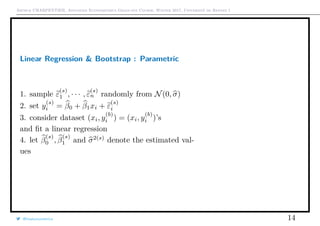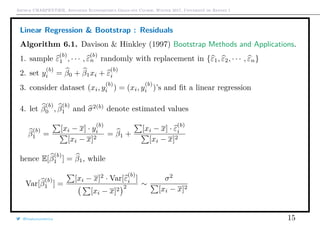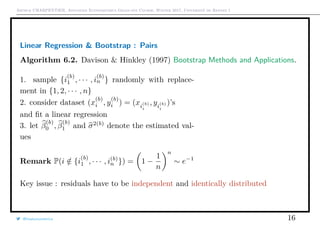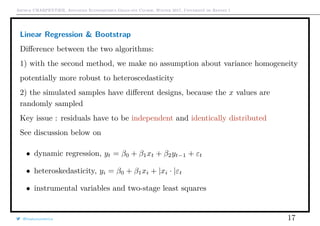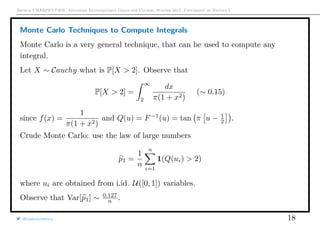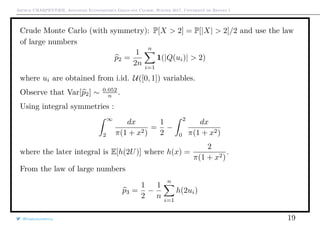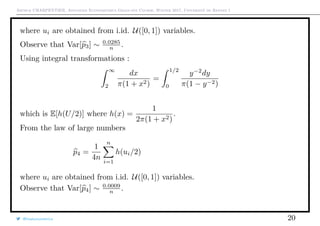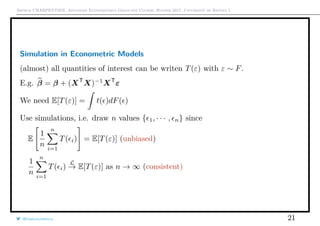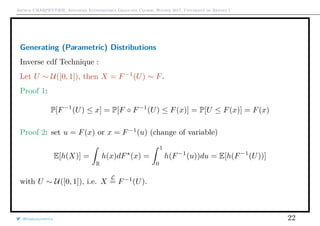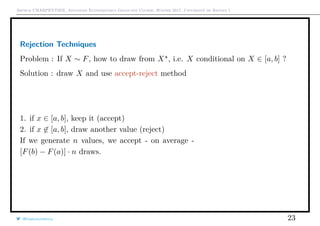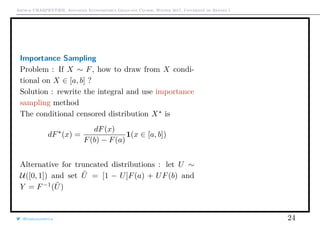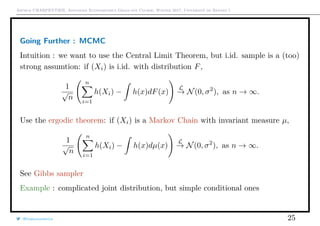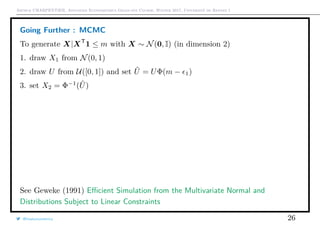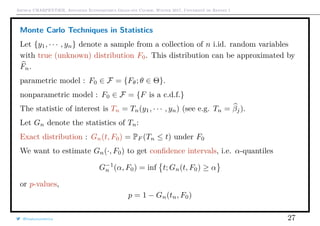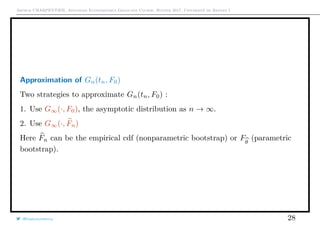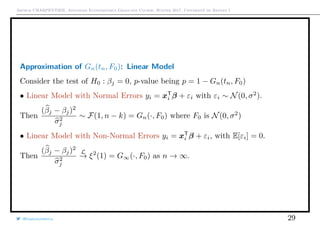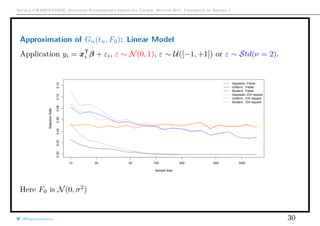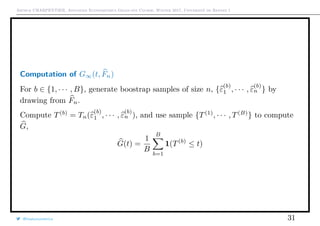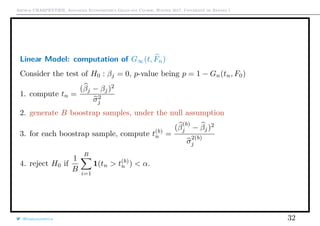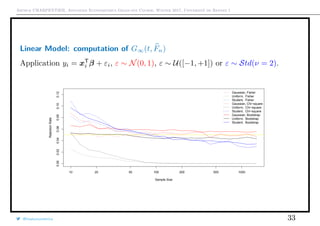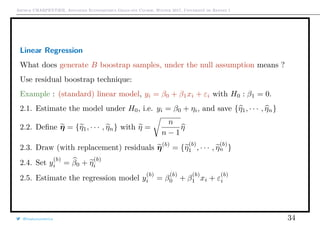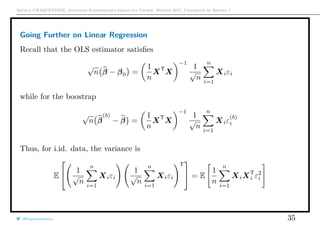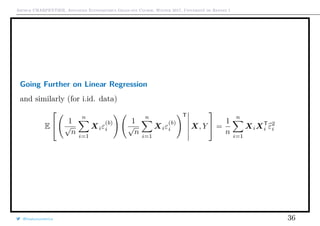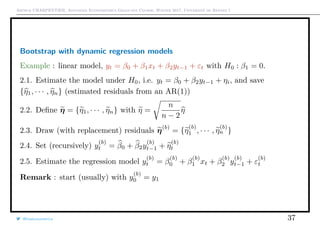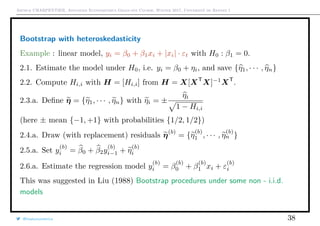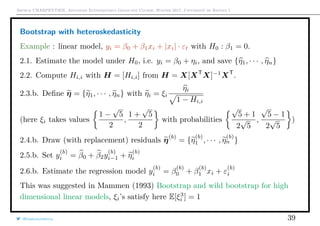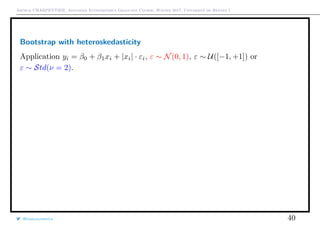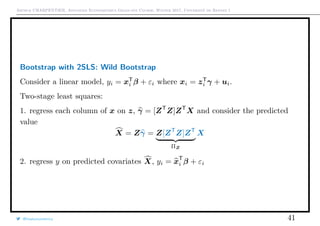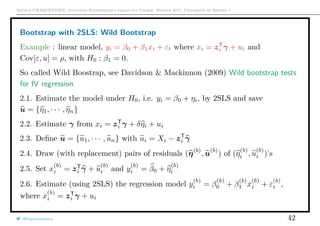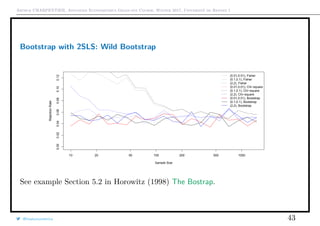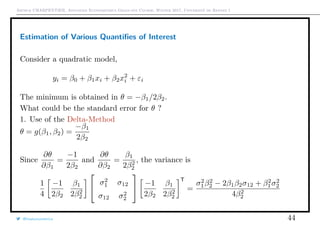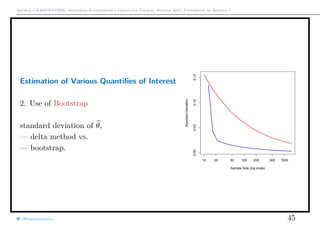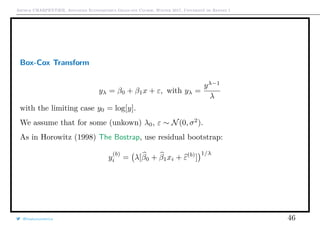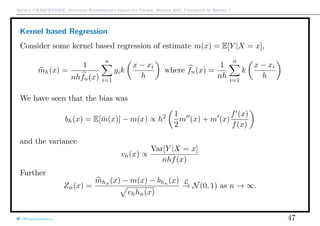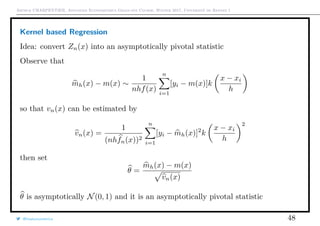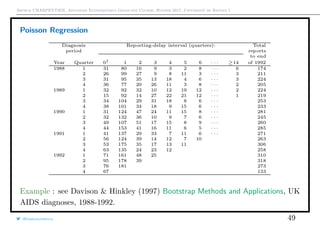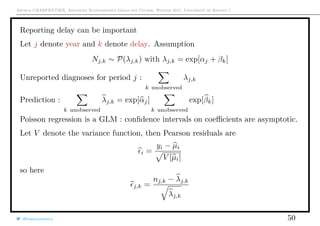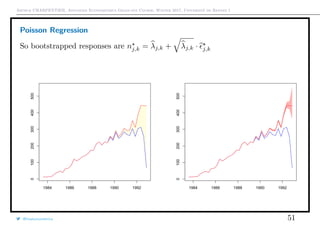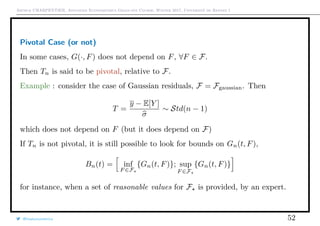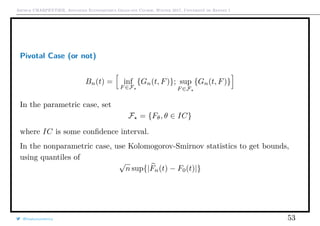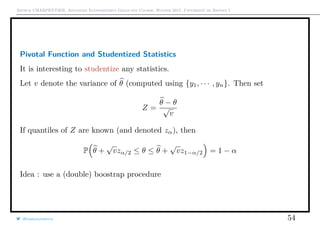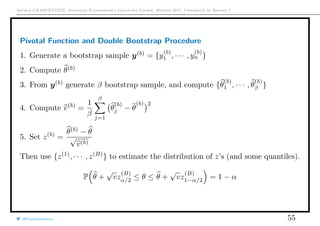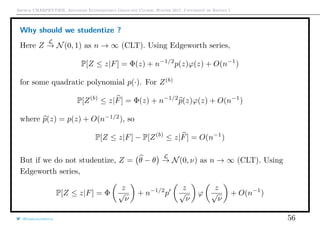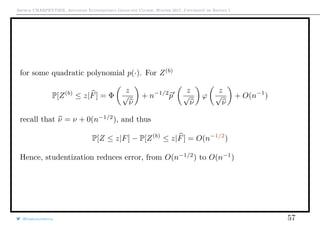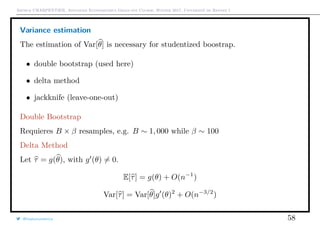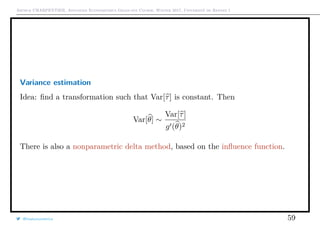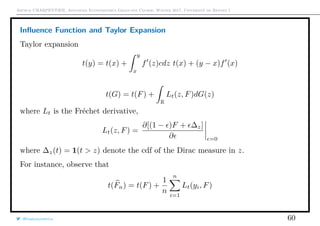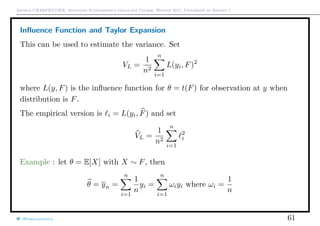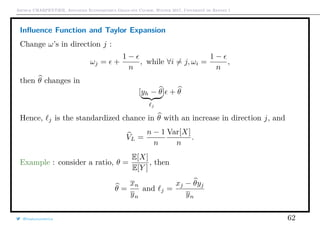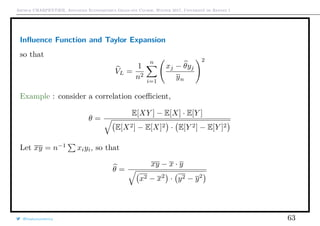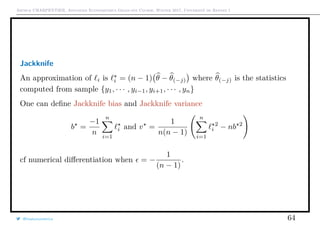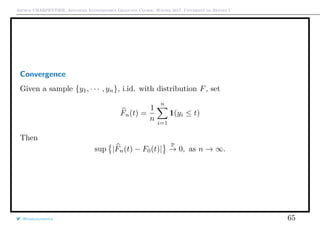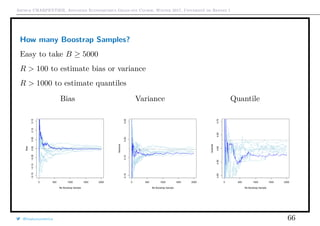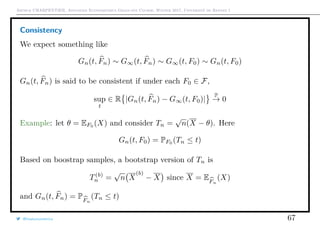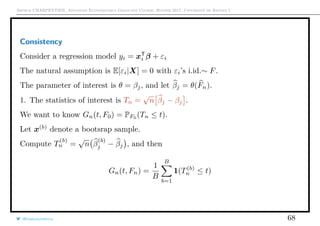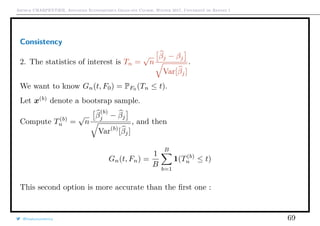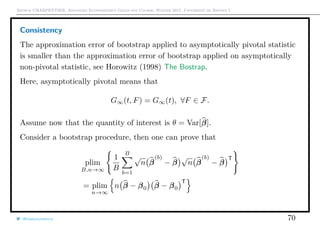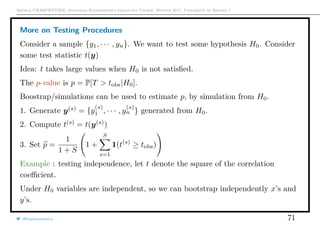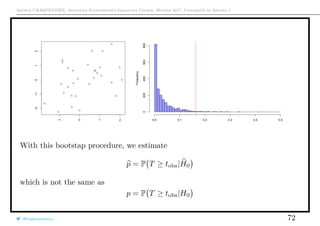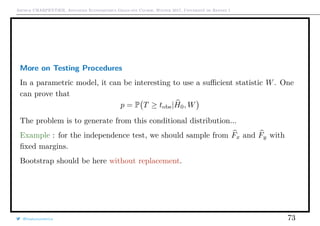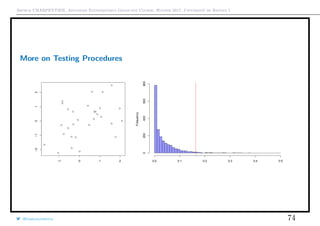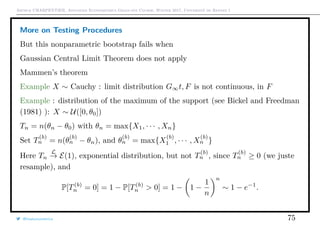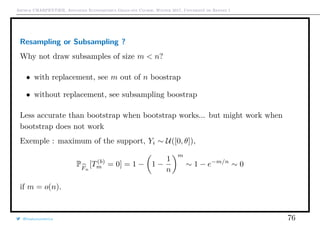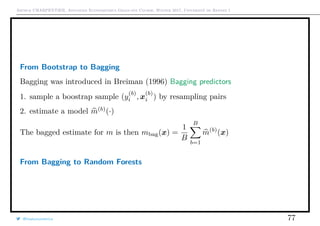1 sur 77
Publicité

1. Arthur CHARPENTIER, Advanced Econometrics Graduate Course, Winter 2017, Université de Rennes 1 Advanced Econometrics #2: Simulations & Bootstrap* A. Charpentier (Université de Rennes 1) Université de Rennes 1, Graduate Course, 2017. @freakonometrics 1
2. Arthur CHARPENTIER, Advanced Econometrics Graduate Course, Winter 2017, Université de Rennes 1 Motivation Before computers, statistical analysis used probability theory to derive statistical expression for standard errors (or conﬁdence intervals) and testing procedures, for some linear model yi = xT i β + εi = β0 + p j=1 βjxj,i + εi. But most formulas are approximations, based on large samples (n → ∞). With computers, simulations and resampling methods can be used to produce (numerical) standard errors and testing procedure (without the use of formulas, but with a simple algorithm). @freakonometrics 2
3. Arthur CHARPENTIER, Advanced Econometrics Graduate Course, Winter 2017, Université de Rennes 1 Overview Linear Regression Model: yi = β0 + xT i β + εi = β0 + β1x1,i + β2x2,i + εi • Nonlinear Transformations : smoothing techniques • Asymptotics vs. Finite Distance : boostrap techniques • Penalization : Parcimony, Complexity and Overﬁt • From least squares to other regressions : quantiles, expectiles, etc. @freakonometrics 3
4. Arthur CHARPENTIER, Advanced Econometrics Graduate Course, Winter 2017, Université de Rennes 1 Historical References Permutation methods go back to Fisher (1935) The Design of Experiments and Pitman (1937) Signiﬁcance tests which may be applied to samples from any population (there are n! distinct permutations) Jackknife was introduced in Quenouille (1949) Approximate tests of correlation in time series, popularized by Tukey (1958) Bias and conﬁdence in not quite large samples Bootstrapping started with Monte Carlo algorithms in the 40’s, see e.g. Simon & Burstein (1969) Basic Research Methods in Social Science Efron (1979) Bootstrap methods: Another look at the jackknife deﬁned a resampling procedure that was coined as “bootstrap”. (there are nn possible distinct ordered bootstrap samples) @freakonometrics 4
5. Arthur CHARPENTIER, Advanced Econometrics Graduate Course, Winter 2017, Université de Rennes 1 References Motivation Bertrand, M., Duﬂo, E. & Mullainathan, 2004. Should we trust diﬀerence-in-diﬀerence estimators?. QJE. References Davison, A.C. & Hinkley, D.V. 1997 Bootstrap Methods and Their Application. CUP. Efron B. & Tibshirani, R.J. An Introduction to the Bootstrap. CRC Press. Horowitz, J.L. 1998 The Bostrap, Handbook of Econometrics, North-Holland. MacKinnon, J. 2007 Bootstrap Hypothesis Testing, Working Paper. @freakonometrics 5
6. Arthur CHARPENTIER, Advanced Econometrics Graduate Course, Winter 2017, Université de Rennes 1 Bootstrap Techniques (in one slide) Bootstrapping is an asymptotic reﬁnement based on computer based simulations. Underlying properties: we know when it might work, or not Idea : {(yi, xi)} is obtained from a stochas- tic model under P We want to generate other samples (not more observations) to reduce uncertainty. @freakonometrics 6 q q
7. Arthur CHARPENTIER, Advanced Econometrics Graduate Course, Winter 2017, Université de Rennes 1 Heuristic Intuition for a Simple (Financial) Model Consider a return stochastic model, rt = µ + σεt, for t = 1, 2, · · · , T, with (εt) is i.id. N(0, 1) [Constant Expected Return Model, CER] µ = 1 T T t=1 rt and σ2 = 1 T T t=1 rt − µ 2 then (standard errors) se[µ] = σ √ T and se[σ] = σ √ 2T then (conﬁdence intervals) µ ∈ µ ± 2se[µ] and σ ∈ σ ± 2se[σ] What if the quantity of interest, θ, is another quantity, e.g. a Value-at-Risk ? @freakonometrics 7
8. Arthur CHARPENTIER, Advanced Econometrics Graduate Course, Winter 2017, Université de Rennes 1 Heuristic Intuition for a Simple (Financial) Model One can use nonparametric bootstrap 1. resampling: generate B “bootstrap samples” by resampling with replacement in the original data, r(b) = {r (b) 1 , · · · , r (b) T }, with r (b) t ∈ {r1, · · · , rT }. 2. For each sample r(b) , compute θ(b) 3. Derive the empirical distribution of θ from θ(1) , · · · , θ(B) . 4. Compute any quantity of interest, standard error, quantiles, etc. E.g. estimate the bias bias[θ] = 1 B B b=1 θ(b) bootstrap mean − 1 B B b=1 θ estimate @freakonometrics 8
9. Arthur CHARPENTIER, Advanced Econometrics Graduate Course, Winter 2017, Université de Rennes 1 Heuristic Intuition for a Simple (Financial) Model E.g. estimate the standard error se[θ] = 1 B − 1 B b=1 θ(b) − 1 B B b=1 θ(b) 2 E.g. estimate the conﬁdence interval, if the bootstrap distribution looks Gaussian θ ∈ θ ± 2se[θ] and if the distribution does not look Gaussian θ ∈ q (B) α/2; q (B) 1−α/2 where q (B) α denote a quantile from θ(1) , · · · , θ(B) . @freakonometrics 9
10. Arthur CHARPENTIER, Advanced Econometrics Graduate Course, Winter 2017, Université de Rennes 1 Monte Carlo Techniques in Statistics Law of large numbers (---), if E[X] = 0 and Var[X] = 1 : √ n Xn L → N(0, 1) What if n is small? What is the distribution of Xn? Example : X such that 2− 1 2 (X − 1) ∼ χ2 (1) Use Monte Carlo Simulation to derive conﬁdence in- tervall for Xn (—). Generate samples {x (m) 1 , · · · , x (m) n } from χ2 (1), and compute x (m) n Then estimate the density of {x (1) n , · · · , x (m) n }, quan- tiles, etc. −0.5 0.0 0.5 0.00.51.01.5 Problem : need to know the true distribution of X. What if we have only {x1, · · · , xn} ? Generate samples {x (m) 1 , · · · , x (m) n } from Fn, and compute x (m) n (—) @freakonometrics 10
11. Arthur CHARPENTIER, Advanced Econometrics Graduate Course, Winter 2017, Université de Rennes 1 Monte Carlo Techniques in Statistics Consider empirical residuals from a linear regres- sion, εi = yi − xT i β. Let F(z) = 1 n n i=1 1 εi σ ≤ z denote the empirical distribution of Studentized residuals. Could we test H0 : F = N(0, 1)? 1 > X <- rnorm (50) 2 > cdf <- function(z) mean(X<=z) Simulate samples from a N(0, 1) (true distribution under H0) @freakonometrics 11 −2 −1 0 1 2 3 0.00.20.40.60.81.0
12. Arthur CHARPENTIER, Advanced Econometrics Graduate Course, Winter 2017, Université de Rennes 1 Quantifying Bias Consider X with mean µ = E(X). Let θ = exp[µ], then θ = exp[x] is a biased estimator of θ, see Horowitz (1998) The Bostrap Idea 1 : Delta Method, i.e. if √ n[τn − τ] L −→ N(0, σ2 ), then, if g (τ) exists and is non-null, √ n[g(τn) − g(τ)] L −→ N(0, σ2 [g (τ)]2 ) so θ1 = exp[x] is asymptotically unbiased. Idea 2 : Delta Method based correction, based on θ2 = exp x − s2 2n where s2 = 1 n n i=1 [xi − x]2 . Idea 3 : Use Bootstrap, θ3 = 1 B n b=1 exp[x(b) ] @freakonometrics 12
13. Arthur CHARPENTIER, Advanced Econometrics Graduate Course, Winter 2017, Université de Rennes 1 Quantifying Bias X with mean µ = E(X). Let θ = exp[µ]. Consider three distributions Log-normal Student t10 Student t5 q q q q q q q q q q q q q q 20 40 60 80 100 120 140 −0.10.00.10.20.3 Sample size q q q q q q q q q q q q q q q q q q q q q q q q q q q q no correction correction second order q q q q q q q q q q q q q q q 20 40 60 80 100 120 140 −0.10.00.10.20.3 Sample sizeq q q q q q q q q q q q q q q q q q q q q q q q q q q q q q no correction correction second order q q q q q q q q q q q q q q 20 40 60 80 100 120 140 −0.10.00.10.20.3 Sample size q q q q q q q q q q q q qq q q q q q q q q q q q q q q no correction correction second order @freakonometrics 13
14. Arthur CHARPENTIER, Advanced Econometrics Graduate Course, Winter 2017, Université de Rennes 1 Linear Regression & Bootstrap : Parametric 1. sample ε (s) 1 , · · · , ε (s) n randomly from N(0, σ) 2. set y (s) i = β0 + β1xi + ε (s) i 3. consider dataset (xi, y (b) i ) = (xi, y (b) i )’s and ﬁt a linear regression 4. let β (s) 0 , β (s) 1 and σ2(s) denote the estimated val- ues @freakonometrics 14 q q q q q q q q q q q q q q q q qq q q q q q q q q q q q q q q q q q q q q q q q q q q q q qq q q 5 10 15 20 25 020406080100120 speed dist
15. Arthur CHARPENTIER, Advanced Econometrics Graduate Course, Winter 2017, Université de Rennes 1 Linear Regression & Bootstrap : Residuals Algorithm 6.1. Davison & Hinkley (1997) Bootstrap Methods and Applications. 1. sample ε (b) 1 , · · · , ε (b) n randomly with replacement in {ε1, ε2, · · · , εn} 2. set y (b) i = β0 + β1xi + ε (b) i 3. consider dataset (xi, y (b) i ) = (xi, y (b) i )’s and ﬁt a linear regression 4. let β (b) 0 , β (b) 1 and σ2(b) denote estimated values β (b) 1 = [xi − x] · y (b) i [xi − x]2 = β1 + [xi − x] · ε (b) i [xi − x]2 hence E[β (b) 1 ] = β1, while Var[β (b) 1 ] = [xi − x]2 · Var[ε (b) i ] [xi − x]2 2 ∼ σ2 [xi − x]2 @freakonometrics 15 q q q q q q q q q q q q q q q q qq q q q q q q q q q q q q q q q q q q q q q q q q q q q q qq q q 5 10 15 20 25 020406080100120 speed dist
16. Arthur CHARPENTIER, Advanced Econometrics Graduate Course, Winter 2017, Université de Rennes 1 Linear Regression & Bootstrap : Pairs Algorithm 6.2. Davison & Hinkley (1997) Bootstrap Methods and Applications. 1. sample {i (b) 1 , · · · , i (b) n } randomly with replace- ment in {1, 2, · · · , n} 2. consider dataset (x (b) i , y (b) i ) = (xi (b) i , yi (b) i )’s and ﬁt a linear regression 3. let β (b) 0 , β (b) 1 and σ2(b) denote the estimated val- ues Remark P(i /∈ {i (b) 1 , · · · , i(b) n }) = 1 − 1 n n ∼ e−1 Key issue : residuals have to be independent and identically distributed @freakonometrics 16 q q q q q q q q q q q q q q q q qq q q q q q q q q q q q q q q q q q q q q q q q q q q q q qq q q 5 10 15 20 25 020406080100120 speed dist
17. Arthur CHARPENTIER, Advanced Econometrics Graduate Course, Winter 2017, Université de Rennes 1 Linear Regression & Bootstrap Diﬀerence between the two algorithms: 1) with the second method, we make no assumption about variance homogeneity potentially more robust to heteroscedasticity 2) the simulated samples have diﬀerent designs, because the x values are randomly sampled Key issue : residuals have to be independent and identically distributed See discussion below on • dynamic regression, yt = β0 + β1xt + β2yt−1 + εt • heteroskedasticity, yi = β0 + β1xi + |xi · |εt • instrumental variables and two-stage least squares @freakonometrics 17
18. Arthur CHARPENTIER, Advanced Econometrics Graduate Course, Winter 2017, Université de Rennes 1 Monte Carlo Techniques to Compute Integrals Monte Carlo is a very general technique, that can be used to compute any integral. Let X ∼ Cauchy what is P[X > 2]. Observe that P[X > 2] = ∞ 2 dx π(1 + x2) (∼ 0.15) since f(x) = 1 π(1 + x2) and Q(u) = F−1 (u) = tan π u − 1 2 . Crude Monte Carlo: use the law of large numbers p1 = 1 n n i=1 1(Q(ui) > 2) where ui are obtained from i.id. U([0, 1]) variables. Observe that Var[p1] ∼ 0.127 n . @freakonometrics 18
19. Arthur CHARPENTIER, Advanced Econometrics Graduate Course, Winter 2017, Université de Rennes 1 Crude Monte Carlo (with symmetry): P[X > 2] = P[|X| > 2]/2 and use the law of large numbers p2 = 1 2n n i=1 1(|Q(ui)| > 2) where ui are obtained from i.id. U([0, 1]) variables. Observe that Var[p2] ∼ 0.052 n . Using integral symmetries : ∞ 2 dx π(1 + x2) = 1 2 − 2 0 dx π(1 + x2) where the later integral is E[h(2U)] where h(x) = 2 π(1 + x2) . From the law of large numbers p3 = 1 2 − 1 n n i=1 h(2ui) @freakonometrics 19
20. Arthur CHARPENTIER, Advanced Econometrics Graduate Course, Winter 2017, Université de Rennes 1 where ui are obtained from i.id. U([0, 1]) variables. Observe that Var[p3] ∼ 0.0285 n . Using integral transformations : ∞ 2 dx π(1 + x2) = 1/2 0 y−2 dy π(1 − y−2) which is E[h(U/2)] where h(x) = 1 2π(1 + x2) . From the law of large numbers p4 = 1 4n n i=1 h(ui/2) where ui are obtained from i.id. U([0, 1]) variables. Observe that Var[p4] ∼ 0.0009 n . @freakonometrics 20 0 2000 4000 6000 8000 10000 0.1350.1400.1450.1500.1550.160 Estimator1
21. Arthur CHARPENTIER, Advanced Econometrics Graduate Course, Winter 2017, Université de Rennes 1 Simulation in Econometric Models (almost) all quantities of interest can be writen T(ε) with ε ∼ F. E.g. β = β + (XT X)−1 XT ε We need E[T(ε)] = t( )dF( ) Use simulations, i.e. draw n values { 1, · · · , n} since E 1 n n i=1 T( i) = E[T(ε)] (unbiased) 1 n n i=1 T( i) L → E[T(ε)] as n → ∞ (consistent) @freakonometrics 21
22. Arthur CHARPENTIER, Advanced Econometrics Graduate Course, Winter 2017, Université de Rennes 1 Generating (Parametric) Distributions Inverse cdf Technique : Let U ∼ U([0, 1]), then X = F−1 (U) ∼ F. Proof 1: P[F−1 (U) ≤ x] = P[F ◦ F−1 (U) ≤ F(x)] = P[U ≤ F(x)] = F(x) Proof 2: set u = F(x) or x = F−1 (u) (change of variable) E[h(X)] = R h(x)dF (x) = 1 0 h(F−1 (u))du = E[h(F−1 (U))] with U ∼ U([0, 1]), i.e. X L = F−1 (U). @freakonometrics 22
23. Arthur CHARPENTIER, Advanced Econometrics Graduate Course, Winter 2017, Université de Rennes 1 Rejection Techniques Problem : If X ∼ F, how to draw from X , i.e. X conditional on X ∈ [a, b] ? Solution : draw X and use accept-reject method 1. if x ∈ [a, b], keep it (accept) 2. if x ∈ [a, b], draw another value (reject) If we generate n values, we accept - on average - [F(b) − F(a)] · n draws. @freakonometrics 23 0 1 2 3 4 5 0.00.20.40.60.81.0
24. Arthur CHARPENTIER, Advanced Econometrics Graduate Course, Winter 2017, Université de Rennes 1 Importance Sampling Problem : If X ∼ F, how to draw from X condi- tional on X ∈ [a, b] ? Solution : rewrite the integral and use importance sampling method The conditional censored distribution X is dF (x) = dF(x) F(b) − F(a) 1(x ∈ [a, b]) Alternative for truncated distributions : let U ∼ U([0, 1]) and set ˜U = [1 − U]F(a) + UF(b) and Y = F−1 ( ˜U) @freakonometrics 24 0 1 2 3 4 5 0.00.20.40.60.81.0 0 1 2 3 4 5 0.00.20.40.60.81.0
25. Arthur CHARPENTIER, Advanced Econometrics Graduate Course, Winter 2017, Université de Rennes 1 Going Further : MCMC Intuition : we want to use the Central Limit Theorem, but i.id. sample is a (too) strong assumtion: if (Xi) is i.id. with distribution F, 1 √ n n i=1 h(Xi) − h(x)dF(x) L → N(0, σ2 ), as n → ∞. Use the ergodic theorem: if (Xi) is a Markov Chain with invariant measure µ, 1 √ n n i=1 h(Xi) − h(x)dµ(x) L → N(0, σ2 ), as n → ∞. See Gibbs sampler Example : complicated joint distribution, but simple conditional ones @freakonometrics 25
26. Arthur CHARPENTIER, Advanced Econometrics Graduate Course, Winter 2017, Université de Rennes 1 Going Further : MCMC To generate X|XT 1 ≤ m with X ∼ N(0, I) (in dimension 2) 1. draw X1 from N(0, 1) 2. draw U from U([0, 1]) and set ˜U = UΦ(m − 1) 3. set X2 = Φ−1 ( ˜U) See Geweke (1991) Eﬃcient Simulation from the Multivariate Normal and Distributions Subject to Linear Constraints @freakonometrics 26 q q q q qq q q q q q q q q q q q q q q q q q q q q q q q q q q q q q q q q q q q q q q q q q q q q q q q q q q q q q q q q q q q q q q q q q q q qq q q q q q q q q q q q q q q q q qq q q q q q q q q q q q q q q q q q q q q q qq q q q q q q q q q q q q q q q q q q q q q q q q q q q q q q q q q q q q q q q q q q q q q q q q q q q q q q q q q q q q q q q q q q q q q q q q q q q q q q q q qq q q −3 −2 −1 0 1 2 −3−2−1012 q qq q q q q q q q qq q q q q q q q q q q q q q q q q q q q q q q q q q q q q q q q q q q q q q q q q q q q q q q q q q q q q q q q q q q q q q qq q q q q q q q q q q q q q q q q qq q qq q q q q q q q q q q q q q q q q q q qq q q q q q q q q q qq q q q q q q q q q q q q q q q q q q q q q q q q q q q q q q q q q q q q q q qq q q q qq q q q q q q q q q q q q q q q q q q q q q q q q q q q q −3 −2 −1 0 1 2 −3−2−1012 q q q q q q q q q q q q q q q q q q q q q q q q q q q q q q q q q q q q q q q q q q q q q q q q q q q q q q q q q q q q q q q q q q q q q q q q q q q q q q q q q q q q qq q q q q q q q q q q q q q q q q q q q q q q q q q q q q q q q q q q q q q q q q q qq q q q q q q q q q q q q q q q q q q q q q q q q q q q q q q q q q q q q q q q q q q q q q q q q q q q q q q q q q q q q q q q q q q q q q q q −3 −2 −1 0 1 2 −3−2−1012
27. Arthur CHARPENTIER, Advanced Econometrics Graduate Course, Winter 2017, Université de Rennes 1 Monte Carlo Techniques in Statistics Let {y1, · · · , yn} denote a sample from a collection of n i.id. random variables with true (unknown) distribution F0. This distribution can be approximated by Fn. parametric model : F0 ∈ F = {Fθ; θ ∈ Θ}. nonparametric model : F0 ∈ F = {F is a c.d.f.} The statistic of interest is Tn = Tn(y1, · · · , yn) (see e.g. Tn = βj). Let Gn denote the statistics of Tn: Exact distribution : Gn(t, F0) = PF (Tn ≤ t) under F0 We want to estimate Gn(·, F0) to get conﬁdence intervals, i.e. α-quantiles G−1 n (α, F0) = inf t; Gn(t, F0) ≥ α or p-values, p = 1 − Gn(tn, F0) @freakonometrics 27
28. Arthur CHARPENTIER, Advanced Econometrics Graduate Course, Winter 2017, Université de Rennes 1 Approximation of Gn(tn, F0) Two strategies to approximate Gn(tn, F0) : 1. Use G∞(·, F0), the asymptotic distribution as n → ∞. 2. Use G∞(·, Fn) Here Fn can be the empirical cdf (nonparametric bootstrap) or Fθ (parametric bootstrap). @freakonometrics 28
29. Arthur CHARPENTIER, Advanced Econometrics Graduate Course, Winter 2017, Université de Rennes 1 Approximation of Gn(tn, F0): Linear Model Consider the test of H0 : βj = 0, p-value being p = 1 − Gn(tn, F0) • Linear Model with Normal Errors yi = xT i β + εi with εi ∼ N(0, σ2 ). Then (βj − βj)2 σ2 j ∼ F(1, n − k) = Gn(·, F0) where F0 is N(0, σ2 ) • Linear Model with Non-Normal Errors yi = xT i β + εi, with E[εi] = 0. Then (βj − βj)2 σ2 j L → ξ2 (1) = G∞(·, F0) as n → ∞. @freakonometrics 29
30. Arthur CHARPENTIER, Advanced Econometrics Graduate Course, Winter 2017, Université de Rennes 1 Approximation of Gn(tn, F0): Linear Model Application yi = xT i β + εi, ε ∼ N(0, 1), ε ∼ U([−1, +1]) or ε ∼ Std(ν = 2). 10 20 50 100 200 500 1000 0.000.020.040.060.080.100.12 Sample Size RejectionRate Gaussian, Fisher Uniform, Fisher Student, Fisher Gaussian, Chi−square Uniform, Chi−square Student, Chi−square Here F0 is N(0, σ2 ) @freakonometrics 30
31. Arthur CHARPENTIER, Advanced Econometrics Graduate Course, Winter 2017, Université de Rennes 1 Computation of G∞(t, Fn) For b ∈ {1, · · · , B}, generate boostrap samples of size n, {ε (b) 1 , · · · , ε (b) n } by drawing from Fn. Compute T(b) = Tn(ε (b) 1 , · · · , ε (b) n ), and use sample {T(1) , · · · , T(B) } to compute G, G(t) = 1 B B b=1 1(T(b) ≤ t) @freakonometrics 31
32. Arthur CHARPENTIER, Advanced Econometrics Graduate Course, Winter 2017, Université de Rennes 1 Linear Model: computation of G∞(t, Fn) Consider the test of H0 : βj = 0, p-value being p = 1 − Gn(tn, F0) 1. compute tn = (βj − βj)2 σ2 j 2. generate B boostrap samples, under the null assumption 3. for each boostrap sample, compute t(b) n = (β (b) j − βj)2 σ 2(b) j 4. reject H0 if 1 B B i=1 1(tn > t(b) n ) < α. @freakonometrics 32
33. Arthur CHARPENTIER, Advanced Econometrics Graduate Course, Winter 2017, Université de Rennes 1 Linear Model: computation of G∞(t, Fn) Application yi = xT i β + εi, ε ∼ N(0, 1), ε ∼ U([−1, +1]) or ε ∼ Std(ν = 2). 10 20 50 100 200 500 1000 0.000.020.040.060.080.100.12 Sample Size RejectionRate Gaussian, Fisher Uniform, Fisher Student, Fisher Gaussian, Chi−square Uniform, Chi−square Student, Chi−square Gaussian, Bootstrap Uniform, Bootstrap Student, Bootstrap @freakonometrics 33
34. Arthur CHARPENTIER, Advanced Econometrics Graduate Course, Winter 2017, Université de Rennes 1 Linear Regression What does generate B boostrap samples, under the null assumption means ? Use residual boostrap technique: Example : (standard) linear model, yi = β0 + β1xi + εi with H0 : β1 = 0. 2.1. Estimate the model under H0, i.e. yi = β0 + ηi, and save {η1, · · · , ηn} 2.2. Deﬁne η = {η1, · · · , ηn} with η = n n − 1 η 2.3. Draw (with replacement) residuals η(b) = {η (b) 1 , · · · , η (b) n } 2.4. Set y (b) i = β0 + η (b) i 2.5. Estimate the regression model y (b) i = β (b) 0 + β (b) 1 xi + ε (b) i @freakonometrics 34
35. Arthur CHARPENTIER, Advanced Econometrics Graduate Course, Winter 2017, Université de Rennes 1 Going Further on Linear Regression Recall that the OLS estimator satisﬁes √ n β − β0 = 1 n XT X −1 1 √ n n i=1 Xiεi while for the boostrap √ n β (b) − β = 1 n XT X −1 1 √ n n i=1 Xiε (b) i Thus, for i.id. data, the variance is E   1 √ n n i=1 Xiεi 1 √ n n i=1 Xiεi T   = E 1 n n i=1 XiXT i ε2 i @freakonometrics 35
36. Arthur CHARPENTIER, Advanced Econometrics Graduate Course, Winter 2017, Université de Rennes 1 Going Further on Linear Regression and similarly (for i.id. data) E   1 √ n n i=1 Xiε (b) i 1 √ n n i=1 Xiε (b) i T X, Y   = 1 n n i=1 XiXT i ε2 i @freakonometrics 36
37. Arthur CHARPENTIER, Advanced Econometrics Graduate Course, Winter 2017, Université de Rennes 1 Bootstrap with dynamic regression models Example : linear model, yt = β0 + β1xt + β2yt−1 + εt with H0 : β1 = 0. 2.1. Estimate the model under H0, i.e. yt = β0 + β2yt−1 + ηi, and save {η1, · · · , ηn} (estimated residuals from an AR(1)) 2.2. Deﬁne η = {η1, · · · , ηn} with η = n n − 2 η 2.3. Draw (with replacement) residuals η(b) = {η (b) 1 , · · · , η (b) n } 2.4. Set (recursively) y (b) t = β0 + β2y (b) t−1 + η (b) t 2.5. Estimate the regression model y (b) t = β (b) 0 + β (b) 1 xt + β (b) 2 y (b) t−1 + ε (b) t Remark : start (usually) with y (b) 0 = y1 @freakonometrics 37
38. Arthur CHARPENTIER, Advanced Econometrics Graduate Course, Winter 2017, Université de Rennes 1 Bootstrap with heteroskedasticity Example : linear model, yi = β0 + β1xi + |xi| · εt with H0 : β1 = 0. 2.1. Estimate the model under H0, i.e. yi = β0 + ηi, and save {η1, · · · , ηn} 2.2. Compute Hi,i with H = [Hi,i] from H = X[XT X]−1 XT . 2.3.a. Deﬁne η = {η1, · · · , ηn} with ηi = ± ηi 1 − Hi,i (here ± mean {−1, +1} with probabilities {1/2, 1/2}) 2.4.a. Draw (with replacement) residuals η(b) = {η (b) 1 , · · · , η (b) n } 2.5.a. Set y (b) i = β0 + β2y (b) i−1 + η (b) i 2.6.a. Estimate the regression model y (b) i = β (b) 0 + β (b) 1 xi + ε (b) i This was suggested in Liu (1988) Bootstrap procedures under some non - i.i.d. models @freakonometrics 38
39. Arthur CHARPENTIER, Advanced Econometrics Graduate Course, Winter 2017, Université de Rennes 1 Bootstrap with heteroskedasticity Example : linear model, yi = β0 + β1xi + |xi| · εt with H0 : β1 = 0. 2.1. Estimate the model under H0, i.e. yi = β0 + ηi, and save {η1, · · · , ηn} 2.2. Compute Hi,i with H = [Hi,i] from H = X[XT X]−1 XT . 2.3.b. Deﬁne η = {η1, · · · , ηn} with ηi = ξi ηi 1 − Hi,i (here ξi takes values 1 − √ 5 2 , 1 + √ 5 2 with probabilities √ 5 + 1 2 √ 5 , √ 5 − 1 2 √ 5 ) 2.4.b. Draw (with replacement) residuals η(b) = {η (b) 1 , · · · , η (b) n } 2.5.b. Set y (b) i = β0 + β2y (b) i−1 + η (b) i 2.6.b. Estimate the regression model y (b) i = β (b) 0 + β (b) 1 xi + ε (b) i This was suggested in Mammen (1993) Bootstrap and wild bootstrap for high dimensional linear models, ξi’s satisfy here E[ξ3 i ] = 1 @freakonometrics 39
40. Arthur CHARPENTIER, Advanced Econometrics Graduate Course, Winter 2017, Université de Rennes 1 Bootstrap with heteroskedasticity Application yi = β0 + β1xi + |xi| · εi, ε ∼ N(0, 1), ε ∼ U([−1, +1]) or ε ∼ Std(ν = 2). @freakonometrics 40 10 20 50 100 200 500 1000 0.000.020.040.060.080.100.12 Sample Size RejectionRate Gaussian, Fisher Uniform, Fisher Student, Fisher Gaussian, Chi−square Uniform, Chi−square Student, Chi−square
41. Arthur CHARPENTIER, Advanced Econometrics Graduate Course, Winter 2017, Université de Rennes 1 Bootstrap with 2SLS: Wild Bootstrap Consider a linear model, yi = xT i β + εi where xi = zT i γ + ui. Two-stage least squares: 1. regress each column of x on z, γ = [ZT Z]ZT X and consider the predicted value X = Zγ = Z[ZT Z]ZT ΠZ X 2. regress y on predicted covariates X, yi = xT i β + εi @freakonometrics 41
42. Arthur CHARPENTIER, Advanced Econometrics Graduate Course, Winter 2017, Université de Rennes 1 Bootstrap with 2SLS: Wild Bootstrap Example : linear model, yi = β0 + β1xi + εt where xi = zT i γ + ui and Cov[ε, u] = ρ, with H0 : β1 = 0. So called Wild Boostrap, see Davidson & Mackinnon (2009) Wild bootstrap tests for IV regression 2.1. Estimate the model under H0, i.e. yi = β0 + ηi, by 2SLS and save u = {η1, · · · , ηn} 2.2. Estimate γ from xi = zT i γ + δηi + ui 2.3. Deﬁne u = {u1, · · · , un} with ui = Xi − zT i γ 2.4. Draw (with replacement) pairs of residuals (η(b) , u(b) ) of (η (b) i , u (b) i )’s 2.5. Set x (b) i = zT i γ + u (b) i and y (b) i = β0 + η (b) i 2.6. Estimate (using 2SLS) the regression model y (b) i = β (b) 0 + β (b) 1 x (b) i + ε (b) i , where x (b) i = zT i γ + ui @freakonometrics 42
43. Arthur CHARPENTIER, Advanced Econometrics Graduate Course, Winter 2017, Université de Rennes 1 Bootstrap with 2SLS: Wild Bootstrap 10 20 50 100 200 500 1000 0.000.020.040.060.080.100.12 Sample Size RejectionRate (0.01,0.01), Fisher (0.1,0.1), Fisher (2,2), Fisher (0.01,0.01), Chi−square (0.1,0.1), Chi−square (2,2), Chi−square (0.01,0.01), Bootstrap (0.1,0.1), Bootstrap (2,2), Bootstrap See example Section 5.2 in Horowitz (1998) The Bostrap. @freakonometrics 43
44. Arthur CHARPENTIER, Advanced Econometrics Graduate Course, Winter 2017, Université de Rennes 1 Estimation of Various Quantiﬁes of Interest Consider a quadratic model, yi = β0 + β1xi + β2x2 i + εi The minimum is obtained in θ = −β1/2β2. What could be the standard error for θ ? 1. Use of the Delta-Method θ = g(β1, β2) = −β1 2β2 Since ∂θ ∂β1 = −1 2β2 and ∂θ ∂β2 = β1 2β2 2 , the variance is 1 4 −1 2β2 β1 2β2 2   σ2 1 σ12 σ12 σ2 2   −1 2β2 β1 2β2 2 T = σ2 1β2 2 − 2β1β2σ12 + β2 1σ2 2 4β2 2 @freakonometrics 44 q q q q q q q q q q qq q q q q q q q q q q q q q q qq q q q q q q q q q qq q q q q q q q q q q q 0.0 0.2 0.4 0.6 0.8 1.0 −1.0−0.50.00.51.0 q
45. Arthur CHARPENTIER, Advanced Econometrics Graduate Course, Winter 2017, Université de Rennes 1 Estimation of Various Quantiﬁes of Interest 2. Use of Bootstrap standard deviation of θ, — delta method vs. — bootstrap. 10 20 50 100 200 500 1000 0.000.050.100.15 Sample Size (log scale) StandardDeviation @freakonometrics 45
46. Arthur CHARPENTIER, Advanced Econometrics Graduate Course, Winter 2017, Université de Rennes 1 Box-Cox Transform yλ = β0 + β1x + ε, with yλ = yλ−1 λ with the limiting case y0 = log[y]. We assume that for some (unkown) λ0, ε ∼ N(0, σ2 ). As in Horowitz (1998) The Bostrap, use residual bootstrap: y (b) i = λ[β0 + β1xi + ε(b) ] 1/λ @freakonometrics 46
47. Arthur CHARPENTIER, Advanced Econometrics Graduate Course, Winter 2017, Université de Rennes 1 Kernel based Regression Consider some kernel based regression of estimate m(x) = E[Y |X = x], mh(x) = 1 nhfn(x) n i=1 yik x − xi h where fn(x) = 1 nh n i=1 k x − xi h We have seen that the bias was bh(x) = E[ ˜m(x)] − m(x) ∝ h2 1 2 m (x) + m (x) f (x) f(x) and the variance vh(x) ∝ Var[Y |X = x] nhf(x) Further Zn(x) = mhn (x) − m(x) − bhn (x) vhhn(x) L → N(0, 1) as n → ∞. @freakonometrics 47
48. Arthur CHARPENTIER, Advanced Econometrics Graduate Course, Winter 2017, Université de Rennes 1 Kernel based Regression Idea: convert Zn(x) into an asymptotically pivotal statistic Observe that mh(x) − m(x) ∼ 1 nhf(x) n i=1 [yi − m(x)]k x − xi h so that vn(x) can be estimated by vn(x) = 1 (nhfn(x))2 n i=1 [yi − mh(x)]2 k x − xi h 2 then set θ = mh(x) − m(x) vn(x) θ is asymptotically N(0, 1) and it is an asymptotically pivotal statistic @freakonometrics 48
49. Arthur CHARPENTIER, Advanced Econometrics Graduate Course, Winter 2017, Université de Rennes 1 Poisson Regression Example : see Davison & Hinkley (1997) Bootstrap Methods and Applications, UK AIDS diagnoses, 1988-1992. @freakonometrics 49
50. Arthur CHARPENTIER, Advanced Econometrics Graduate Course, Winter 2017, Université de Rennes 1 Reporting delay can be important Let j denote year and k denote delay. Assumption Nj,k ∼ P(λj,k) with λj,k = exp[αj + βk] Unreported diagnoses for period j : k unobserved λj,k Prediction : k unobserved λj,k = exp[αj] k unobserved exp[βk] Poisson regression is a GLM : conﬁdence intervals on coeﬃcients are asymptotic. Let V denote the variance function, then Pearson residuals are i = yi − µi V [µi] so here j,k = nj,k − λj,k λj,k @freakonometrics 50
51. Arthur CHARPENTIER, Advanced Econometrics Graduate Course, Winter 2017, Université de Rennes 1 Poisson Regression So bootstrapped responses are nj,k = λj,k + λj,k · j,k 1984 1986 1988 1990 1992 0100200300400500 1984 1986 1988 1990 1992 0100200300400500 @freakonometrics 51
52. Arthur CHARPENTIER, Advanced Econometrics Graduate Course, Winter 2017, Université de Rennes 1 Pivotal Case (or not) In some cases, G(·, F) does not depend on F, ∀F ∈ F. Then Tn is said to be pivotal, relative to F. Example : consider the case of Gaussian residuals, F = Fgaussian. Then T = y − E[Y ] σ ∼ Std(n − 1) which does not depend on F (but it does depend on F) If Tn is not pivotal, it is still possible to look for bounds on Gn(t, F), Bn(t) = inf F ∈F {Gn(t, F)}; sup F ∈F {Gn(t, F)} for instance, when a set of reasonable values for F is provided, by an expert. @freakonometrics 52
53. Arthur CHARPENTIER, Advanced Econometrics Graduate Course, Winter 2017, Université de Rennes 1 Pivotal Case (or not) Bn(t) = inf F ∈F {Gn(t, F)}; sup F ∈F {Gn(t, F)} In the parametric case, set F = {Fθ, θ ∈ IC} where IC is some conﬁdence interval. In the nonparametric case, use Kolomogorov-Smirnov statistics to get bounds, using quantiles of √ n sup{|Fn(t) − F0(t)|} @freakonometrics 53
54. Arthur CHARPENTIER, Advanced Econometrics Graduate Course, Winter 2017, Université de Rennes 1 Pivotal Function and Studentized Statistics It is interesting to studentize any statistics. Let v denote the variance of θ (computed using {y1, · · · , yn}. Then set Z = θ − θ √ v If quantiles of Z are known (and denoted zα), then P θ + √ vzα/2 ≤ θ ≤ θ + √ vz1−α/2 = 1 − α Idea : use a (double) boostrap procedure @freakonometrics 54
55. Arthur CHARPENTIER, Advanced Econometrics Graduate Course, Winter 2017, Université de Rennes 1 Pivotal Function and Double Bootstrap Procedure 1. Generate a bootstrap sample y(b) = {y (b) 1 , · · · , y (b) n } 2. Compute θ(b) 3. From y(b) generate β bootstrap sample, and compute {θ (b) 1 , · · · , θ (b) β } 4. Compute v(b) = 1 β β j=1 θ (b) j − θ (b) 2 5. Set z(b) = θ(b) − θ √ v(b) Then use {z(1) , · · · , z(B) } to estimate the distribution of z’s (and some quantiles). P θ + √ vz (B) α/2 ≤ θ ≤ θ + √ vz (B) 1−α/2 = 1 − α @freakonometrics 55
56. Arthur CHARPENTIER, Advanced Econometrics Graduate Course, Winter 2017, Université de Rennes 1 Why should we studentize ? Here Z L → N(0, 1) as n → ∞ (CLT). Using Edgeworth series, P[Z ≤ z|F] = Φ(z) + n−1/2 p(z)ϕ(z) + O(n−1 ) for some quadratic polynomial p(·). For Z(b) P[Z(b) ≤ z|F] = Φ(z) + n−1/2 p(z)ϕ(z) + O(n−1 ) where p(z) = p(z) + O(n−1/2 ), so P[Z ≤ z|F] − P[Z(b) ≤ z|F] = O(n−1 ) But if we do not studentize, Z = θ − θ L → N(0, ν) as n → ∞ (CLT). Using Edgeworth series, P[Z ≤ z|F] = Φ z √ ν + n−1/2 p z √ ν ϕ z √ ν + O(n−1 ) @freakonometrics 56
57. Arthur CHARPENTIER, Advanced Econometrics Graduate Course, Winter 2017, Université de Rennes 1 for some quadratic polynomial p(·). For Z(b) P[Z(b) ≤ z|F] = Φ z √ ν + n−1/2 p z √ ν ϕ z √ ν + O(n−1 ) recall that ν = ν + 0(n−1/2 ), and thus P[Z ≤ z|F] − P[Z(b) ≤ z|F] = O(n−1/2 ) Hence, studentization reduces error, from O(n−1/2 ) to O(n−1 ) @freakonometrics 57
58. Arthur CHARPENTIER, Advanced Econometrics Graduate Course, Winter 2017, Université de Rennes 1 Variance estimation The estimation of Var[θ] is necessary for studentized boostrap. • double bootstrap (used here) • delta method • jackknife (leave-one-out) Double Bootstrap Requieres B × β resamples, e.g. B ∼ 1, 000 while β ∼ 100 Delta Method Let τ = g(θ), with g (θ) = 0. E[τ] = g(θ) + O(n−1 ) Var[τ] = Var[θ]g (θ)2 + O(n−3/2 ) @freakonometrics 58
59. Arthur CHARPENTIER, Advanced Econometrics Graduate Course, Winter 2017, Université de Rennes 1 Variance estimation Idea: ﬁnd a transformation such that Var[τ] is constant. Then Var[θ] ∼ Var[τ] g (θ)2 There is also a nonparametric delta method, based on the inﬂuence function. @freakonometrics 59
60. Arthur CHARPENTIER, Advanced Econometrics Graduate Course, Winter 2017, Université de Rennes 1 Inﬂuence Function and Taylor Expansion Taylor expansion t(y) = t(x) + y x f (z)cdz t(x) + (y − x)f (x) t(G) = t(F) + R Lt(z, F)dG(z) where Lt is the Fréchet derivative, Lt(z, F) = ∂[(1 − )F + ∆z] ∂ =0 where ∆z(t) = 1(t > z) denote the cdf of the Dirac measure in z. For instance, observe that t(Fn) = t(F) + 1 n n i=1 Lt(yi, F) @freakonometrics 60
61. Arthur CHARPENTIER, Advanced Econometrics Graduate Course, Winter 2017, Université de Rennes 1 Inﬂuence Function and Taylor Expansion This can be used to estimate the variance. Set VL = 1 n2 n i=1 L(yi, F)2 where L(y, F) is the inﬂuence function for θ = t(F) for observation at y when distribution is F. The empirical version is i = L(yi, F) and set VL = 1 n2 n i=1 2 i Example : let θ = E[X] with X ∼ F, then θ = yn = n i=1 1 n yi = n i=1 ωiyi where ωi = 1 n @freakonometrics 61
62. Arthur CHARPENTIER, Advanced Econometrics Graduate Course, Winter 2017, Université de Rennes 1 Inﬂuence Function and Taylor Expansion Change ω’s in direction j : ωj = + 1 − n , while ∀i = j, ωi = 1 − n , then θ changes in [yh − θ j ] + θ Hence, j is the standardized chance in θ with an increase in direction j, and VL = n − 1 n Var[X] n . Example : consider a ratio, θ = E[X] E[Y ] , then θ = xn yn and j = xj − θyj yn @freakonometrics 62
63. Arthur CHARPENTIER, Advanced Econometrics Graduate Course, Winter 2017, Université de Rennes 1 Inﬂuence Function and Taylor Expansion so that VL = 1 n2 n i=1 xj − θyj yn 2 Example : consider a correlation coeﬃcient, θ = E[XY ] − E[X] · E[Y ] E[X2] − E[X]2 · E[Y 2] − E[Y ]2 Let xy = n−1 xiyi, so that θ = xy − x · y x2 − x2 · y2 − y2 @freakonometrics 63
64. Arthur CHARPENTIER, Advanced Econometrics Graduate Course, Winter 2017, Université de Rennes 1 Jackknife An approximation of i is i = (n − 1) θ − θ(−j) where θ(−j) is the statistics computed from sample {y1, · · · , yi−1, yi+1, · · · , yn} One can deﬁne Jackknife bias and Jackknife variance b = −1 n n i=1 i and v = 1 n(n − 1) n i=1 2 i − nb 2 cf numerical diﬀerentiation when = − 1 (n − 1) . @freakonometrics 64
65. Arthur CHARPENTIER, Advanced Econometrics Graduate Course, Winter 2017, Université de Rennes 1 Convergence Given a sample {y1, · · · , yn}, i.id. with distribution F, set Fn(t) = 1 n n i=1 1(yi ≤ t) Then sup |Fn(t) − F0(t)| P → 0, as n → ∞. @freakonometrics 65
66. Arthur CHARPENTIER, Advanced Econometrics Graduate Course, Winter 2017, Université de Rennes 1 How many Boostrap Samples? Easy to take B ≥ 5000 R > 100 to estimate bias or variance R > 1000 to estimate quantiles Bias Variance Quantile 0 500 1000 1500 2000 −0.15−0.10−0.050.000.050.100.15 Nb Boostrap Sample Bias 0 500 1000 1500 2000 0.100.150.200.25 Nb Boostrap Sample Variance 0 500 1000 1500 2000 4.504.554.604.654.70 Nb Boostrap Sample Quantile @freakonometrics 66
67. Arthur CHARPENTIER, Advanced Econometrics Graduate Course, Winter 2017, Université de Rennes 1 Consistency We expect something like Gn(t, Fn) ∼ G∞(t, Fn) ∼ G∞(t, F0) ∼ Gn(t, F0) Gn(t, Fn) is said to be consistent if under each F0 ∈ F, sup t ∈ R |Gn(t, Fn) − G∞(t, F0)| P → 0 Example: let θ = EF0 (X) and consider Tn = √ n(X − θ). Here Gn(t, F0) = PF0 (Tn ≤ t) Based on boostrap samples, a bootstrap version of Tn is T(b) n = √ n X (b) − X since X = EFn (X) and Gn(t, Fn) = PFn (Tn ≤ t) @freakonometrics 67
68. Arthur CHARPENTIER, Advanced Econometrics Graduate Course, Winter 2017, Université de Rennes 1 Consistency Consider a regression model yi = xT i β + εi The natural assumption is E[εi|X] = 0 with εi’s i.id.∼ F. The parameter of interest is θ = βj, and let βj = θ(Fn). 1. The statistics of interest is Tn = √ n βj − βj . We want to know Gn(t, F0) = PF0 (Tn ≤ t). Let x(b) denote a bootsrap sample. Compute T (b) n = √ n β (b) j − βj , and then Gn(t, Fn) = 1 B B b=1 1(T(b) n ≤ t) @freakonometrics 68
69. Arthur CHARPENTIER, Advanced Econometrics Graduate Course, Winter 2017, Université de Rennes 1 Consistency 2. The statistics of interest is Tn = √ n βj − βj Var[βj] . We want to know Gn(t, F0) = PF0 (Tn ≤ t). Let x(b) denote a bootsrap sample. Compute T(b) n = √ n β (b) j − βj Var(b) [βj] , and then Gn(t, Fn) = 1 B B b=1 1(T(b) n ≤ t) This second option is more accurate than the ﬁrst one : @freakonometrics 69
70. Arthur CHARPENTIER, Advanced Econometrics Graduate Course, Winter 2017, Université de Rennes 1 Consistency The approximation error of bootstrap applied to asymptotically pivotal statistic is smaller than the approximation error of bootstrap applied on asymptotically non-pivotal statistic, see Horowitz (1998) The Bostrap. Here, asymptotically pivotal means that G∞(t, F) = G∞(t), ∀F ∈ F. Assume now that the quantity of interest is θ = Var[β]. Consider a bootstrap procedure, then one can prove that plim B,n→∞ 1 B B b=1 √ n β (b) − β √ n β (b) − β T = plim n→∞ n β − β0 β − β0 T @freakonometrics 70
71. Arthur CHARPENTIER, Advanced Econometrics Graduate Course, Winter 2017, Université de Rennes 1 More on Testing Procedures Consider a sample {y1, · · · , yn}. We want to test some hypothesis H0. Consider some test statistic t(y) Idea: t takes large values when H0 is not satisﬁed. The p-value is p = P[T > tobs|H0]. Boostrap/simulations can be used to estimate p, by simulation from H0. 1. Generate y(s) = {y (s) 1 , · · · , y (s) n } generated from H0. 2. Compute t(s) = t(y(s) ) 3. Set p = 1 1 + S 1 + S s=1 1(t(s) ≥ tobs) Example : testing independence, let t denote the square of the correlation coeﬃcient. Under H0 variables are independent, so we can bootstrap independently x’s and y’s. @freakonometrics 71
72. Arthur CHARPENTIER, Advanced Econometrics Graduate Course, Winter 2017, Université de Rennes 1 q q q q q q q q q q q q q q qq q q q q q q q q q q q q q q −1 0 1 2 −2−1012 Frequency 0.0 0.1 0.2 0.3 0.4 0.5 0200400600800 With this bootstap procedure, we estimate p = P T ≥ tobs|H0 which is not the same as p = P T ≥ tobs|H0 @freakonometrics 72
73. Arthur CHARPENTIER, Advanced Econometrics Graduate Course, Winter 2017, Université de Rennes 1 More on Testing Procedures In a parametric model, it can be interesting to use a suﬃcient statistic W. One can prove that p = P T ≥ tobs|H0, W The problem is to generate from this conditional distribution... Example : for the independence test, we should sample from Fx and Fy with ﬁxed margins. Bootstrap should be here without replacement. @freakonometrics 73
74. Arthur CHARPENTIER, Advanced Econometrics Graduate Course, Winter 2017, Université de Rennes 1 More on Testing Procedures q q q q q q q q q q q q q q qq q q q q q q q q q q q q q q −1 0 1 2 −2−1012 Frequency 0.0 0.1 0.2 0.3 0.4 0.5 0200400600800 @freakonometrics 74
75. Arthur CHARPENTIER, Advanced Econometrics Graduate Course, Winter 2017, Université de Rennes 1 More on Testing Procedures But this nonparametric bootstrap fails when Gaussian Central Limit Theorem does not apply Mammen’s theorem Example X ∼ Cauchy : limit distribution G∞t, F is not continuous, in F Example : distribution of the maximum of the support (see Bickel and Freedman (1981) ): X ∼ U([0, θ0]) Tn = n(θn − θ0) with θn = max{X1, · · · , Xn} Set T (b) n = n(θ (b) n − θn), and θ (b) n = max{X (b) 1 , · · · , X (b) n } Here Tn L → E(1), exponential distribution, but not T (b) n , since T (b) n ≥ 0 (we juste resample), and P[T(b) n = 0] = 1 − P[T(b) n > 0] = 1 − 1 − 1 n n ∼ 1 − e−1 . @freakonometrics 75
76. Arthur CHARPENTIER, Advanced Econometrics Graduate Course, Winter 2017, Université de Rennes 1 Resampling or Subsampling ? Why not draw subsamples of size m < n? • with replacement, see m out of n boostrap • without replacement, see subsampling boostrap Less accurate than bootstrap when bootstrap works... but might work when bootstrap does not work Exemple : maximum of the support, Yi ∼ U([0, θ]), PFn [T(b) m = 0] = 1 − 1 − 1 n m ∼ 1 − e−m/n ∼ 0 if m = o(n). @freakonometrics 76
77. Arthur CHARPENTIER, Advanced Econometrics Graduate Course, Winter 2017, Université de Rennes 1 From Bootstrap to Bagging Bagging was introduced in Breiman (1996) Bagging predictors 1. sample a boostrap sample (y (b) i , x (b) i ) by resampling pairs 2. estimate a model m(b) (·) The bagged estimate for m is then mbag(x) = 1 B B b=1 m(b) (x) From Bagging to Random Forests @freakonometrics 77
Publicité